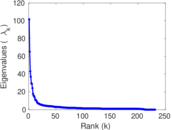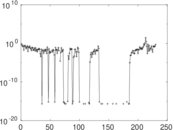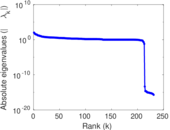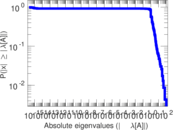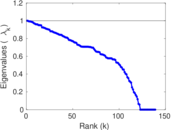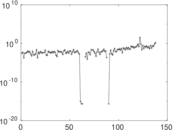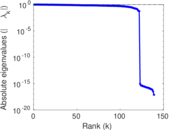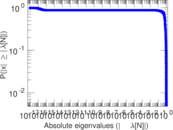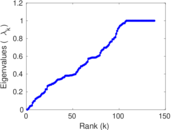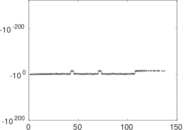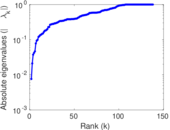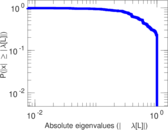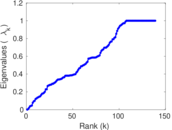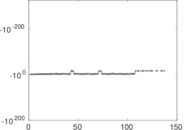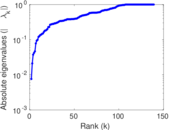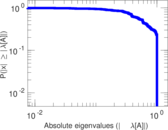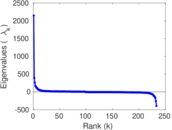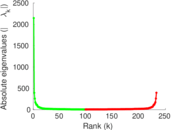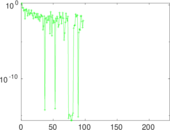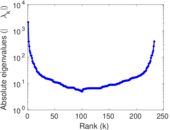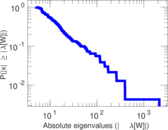# Wikibooks edits (mk)

This is the bipartite edit network of the Macedonian Wikibooks. It contains users and pages from the Macedonian Wikibooks, connected by edit events. Each edge represents an edit. The dataset includes the timestamp of each edit.

 Code `bmk` Internal name `edit-mkwikibooks` Name Wikibooks edits (mk) Data source http://dumps.wikimedia.org/ AvailabilityDataset is available for download Consistency checkDataset passed all tests Category Authorship network Dataset timestamp 2017-10-20 Node meaning User, article Edge meaning Edit Network formatBipartite, undirected Edge typeUnweighted, multiple edges Temporal dataEdges are annotated with timestamps

## Statistics

 Size n = 2,155 Left size n1 = 233 Right size n2 = 1,922 Volume m = 3,748 Unique edge count m̿ = 2,373 Wedge count s = 261,641 Claw count z = 36,005,617 Cross count x = 4,531,369,330 Square count q = 15,826 4-Tour count T4 = 1,178,002 Maximum degree dmax = 1,087 Maximum left degree d1max = 1,087 Maximum right degree d2max = 73 Average degree d = 3.478 42 Average left degree d1 = 16.085 8 Average right degree d2 = 1.950 05 Fill p = 0.005 298 93 Average edge multiplicity m̃ = 1.579 44 Size of LCC N = 1,742 Diameter δ = 17 50-Percentile effective diameter δ0.5 = 3.727 22 90-Percentile effective diameter δ0.9 = 7.355 73 Median distance δM = 4 Mean distance δm = 4.728 23 Gini coefficient G = 0.668 289 Balanced inequality ratio P = 0.245 998 Left balanced inequality ratio P1 = 0.126 734 Right balanced inequality ratio P2 = 0.354 322 Relative edge distribution entropy Her = 0.794 065 Power law exponent γ = 4.780 34 Tail power law exponent γt = 2.571 00 Tail power law exponent with p γ3 = 2.571 00 p-value p = 0.000 00 Left tail power law exponent with p γ3,1 = 1.811 00 Left p-value p1 = 0.057 000 0 Right tail power law exponent with p γ3,2 = 4.931 00 Right p-value p2 = 0.001 000 00 Degree assortativity ρ = −0.172 760 Degree assortativity p-value pρ = 2.349 02 × 10−17 Spectral norm α = 101.686 Algebraic connectivity a = 0.007 582 34 Spectral separation |λ1[A] / λ2[A]| = 1.559 79 Controllability C = 1,598 Relative controllability Cr = 0.789 526

## Plots

### Fruchterman–Reingold graph drawing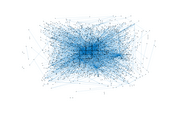### Degree distribution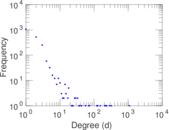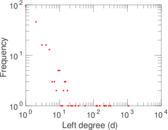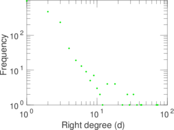### Cumulative degree distribution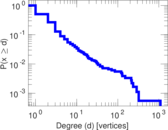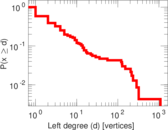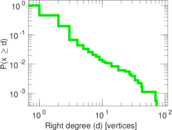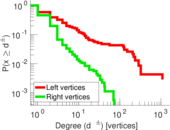### Lorenz curve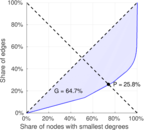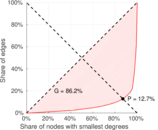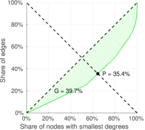### Spectral distribution of the adjacency matrix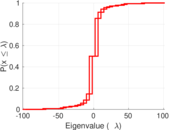### Spectral distribution of the normalized adjacency matrix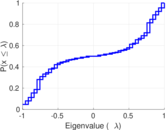### Spectral distribution of the Laplacian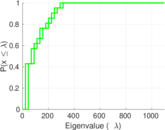### Spectral graph drawing based on the adjacency matrix### Spectral graph drawing based on the Laplacian### Spectral graph drawing based on the normalized adjacency matrix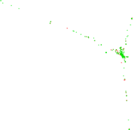### Degree assortativity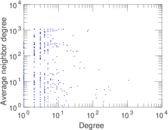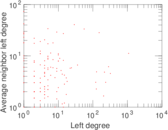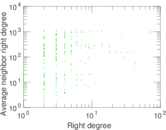### Zipf plot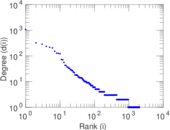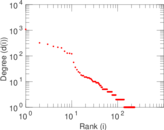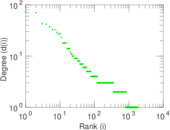### Hop distribution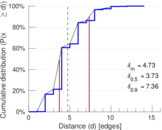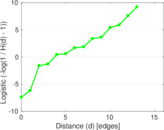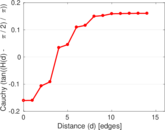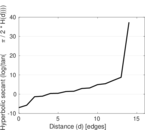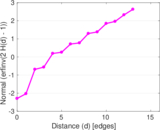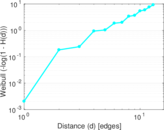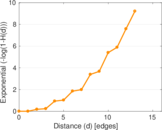### Double Laplacian graph drawing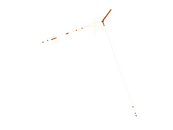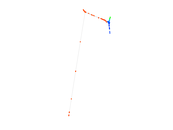### Delaunay graph drawing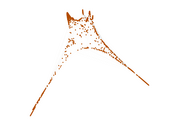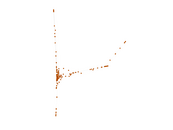### Edge weight/multiplicity distribution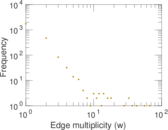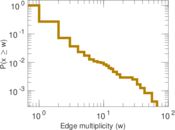### Temporal distribution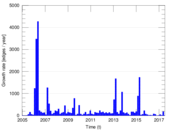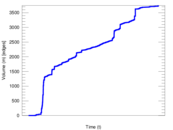### Temporal hop distribution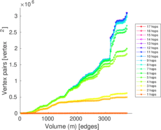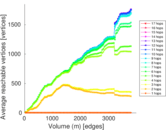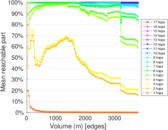### Diameter/density evolution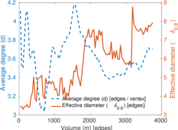### Matrix decompositions plots